Скачать презентацию Intelligent Text Processing lecture 1 Intro to string

33cc7606535612114a4b5dfff446bd7a.ppt

• Количество слайдов: 21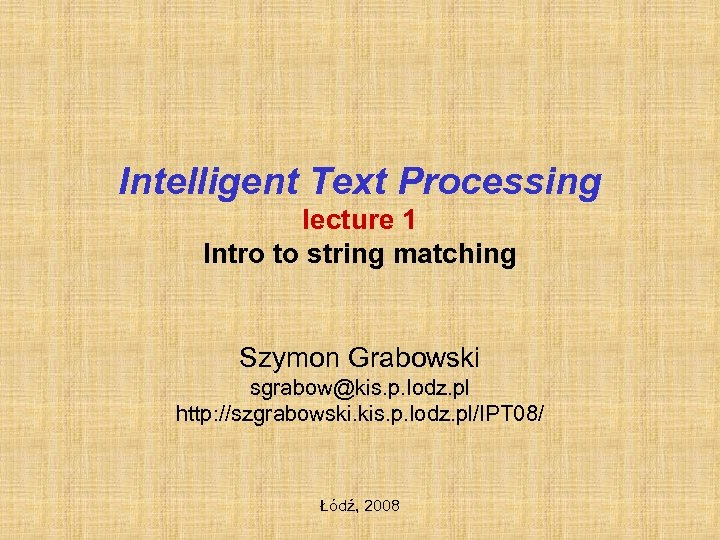Intelligent Text Processing lecture 1 Intro to string matching Szymon Grabowski [email protected] p. lodz. pl http: //szgrabowski. kis. p. lodz. pl/IPT 08/ Łódź, 2008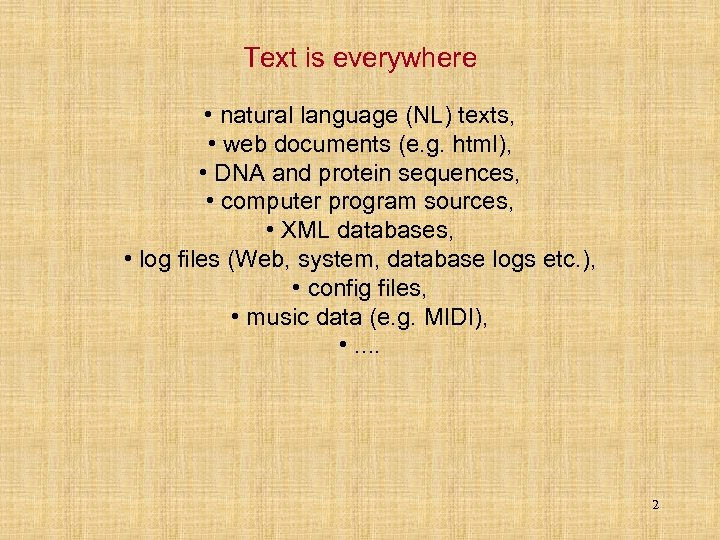Text is everywhere • natural language (NL) texts, • web documents (e. g. html), • DNA and protein sequences, • computer program sources, • XML databases, • log files (Web, system, database logs etc. ), • config files, • music data (e. g. MIDI), • . . 2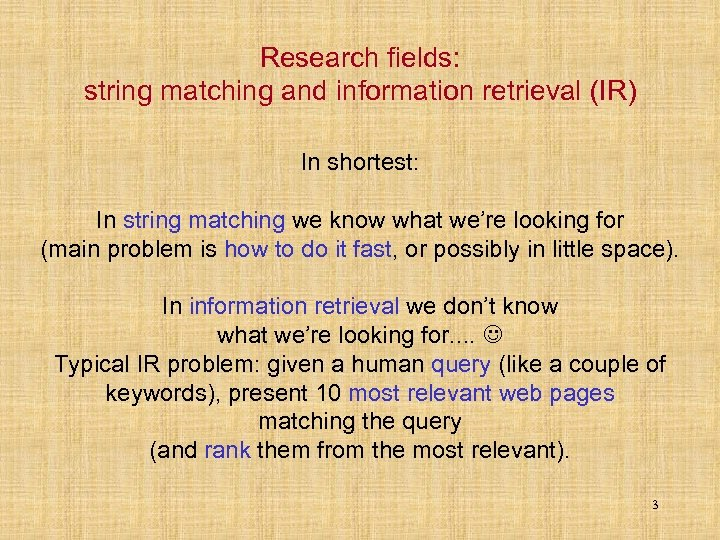Research fields: string matching and information retrieval (IR) In shortest: In string matching we know what we’re looking for (main problem is how to do it fast, or possibly in little space). In information retrieval we don’t know what we’re looking for. . Typical IR problem: given a human query (like a couple of keywords), present 10 most relevant web pages matching the query (and rank them from the most relevant). 3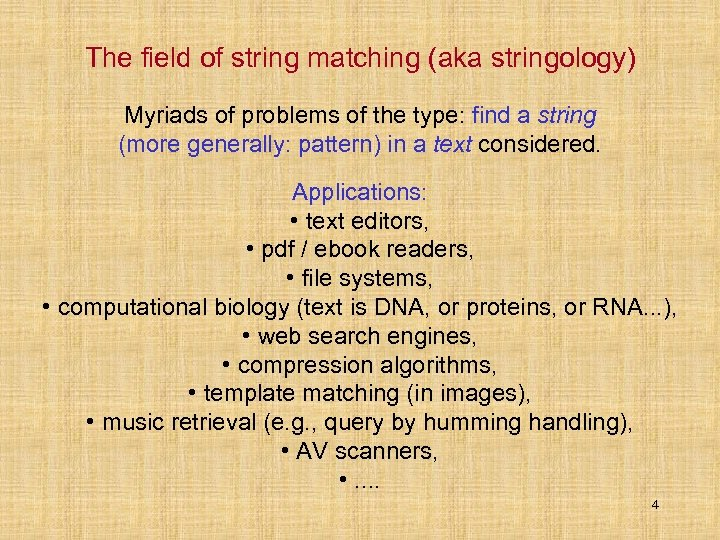The field of string matching (aka stringology) Myriads of problems of the type: find a string (more generally: pattern) in a text considered. Applications: • text editors, • pdf / ebook readers, • file systems, • computational biology (text is DNA, or proteins, or RNA. . . ), • web search engines, • compression algorithms, • template matching (in images), • music retrieval (e. g. , query by humming handling), • AV scanners, • . . 4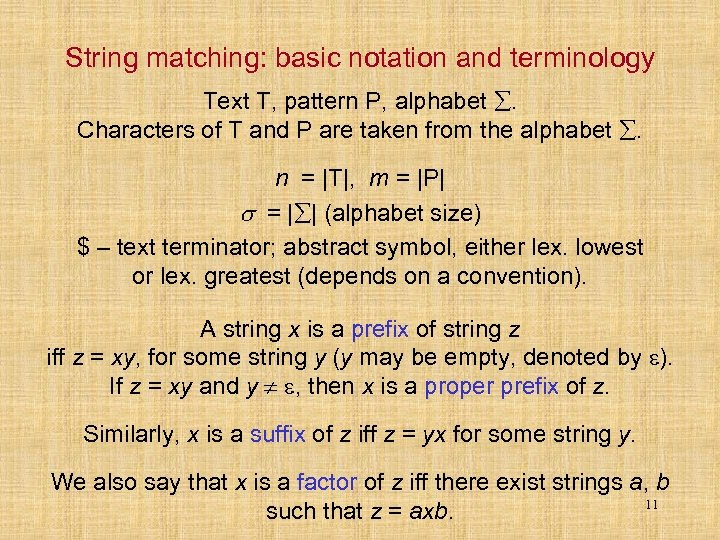String matching: basic notation and terminology Text T, pattern P, alphabet . Characters of T and P are taken from the alphabet . n = |T|, m = |P| = | | (alphabet size) \$ – text terminator; abstract symbol, either lex. lowest or lex. greatest (depends on a convention). A string x is a prefix of string z iff z = xy, for some string y (y may be empty, denoted by ). If z = xy and y , then x is a proper prefix of z. Similarly, x is a suffix of z iff z = yx for some string y. We also say that x is a factor of z iff there exist strings a, b 11 such that z = axb.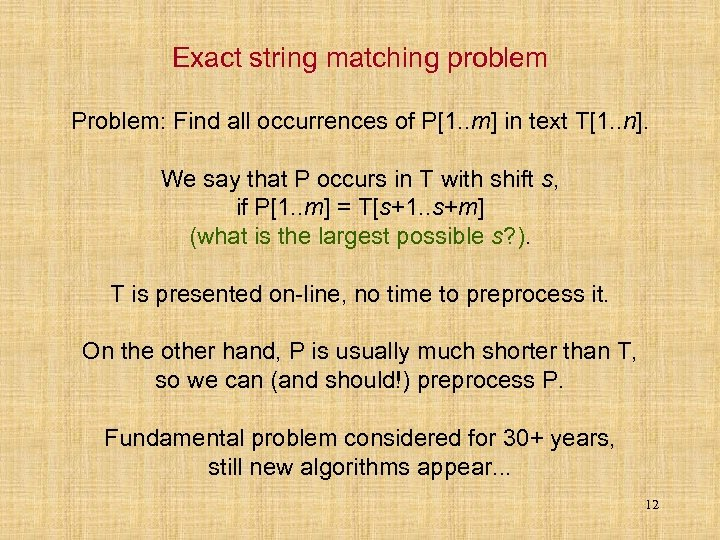Exact string matching problem Problem: Find all occurrences of P[1. . m] in text T[1. . n]. We say that P occurs in T with shift s, if P[1. . m] = T[s+1. . s+m] (what is the largest possible s? ). T is presented on-line, no time to preprocess it. On the other hand, P is usually much shorter than T, so we can (and should!) preprocess P. Fundamental problem considered for 30+ years, still new algorithms appear. . . 12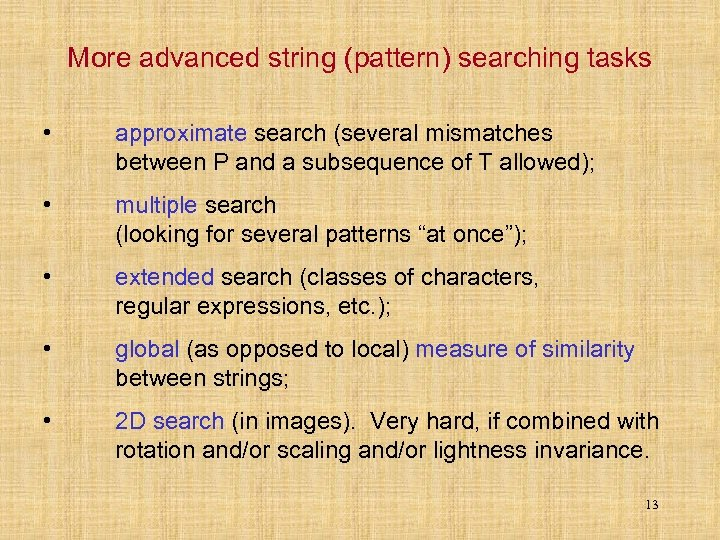More advanced string (pattern) searching tasks • approximate search (several mismatches between P and a subsequence of T allowed); • multiple search (looking for several patterns “at once”); • extended search (classes of characters, regular expressions, etc. ); • global (as opposed to local) measure of similarity between strings; • 2 D search (in images). Very hard, if combined with rotation and/or scaling and/or lightness invariance. 13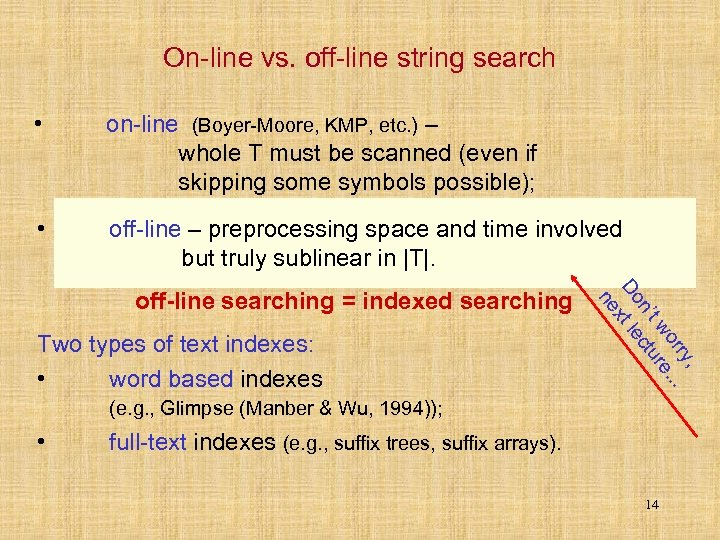On-line vs. off-line string search • on-line (Boyer-Moore, KMP, etc. ) – whole T must be scanned (even if skipping some symbols possible); • off-line – preprocessing space and time involved but truly sublinear in |T|. Two types of text indexes: • word based indexes , ry or e. . . tw r n’ ctu Do xt le ne off-line searching = indexed searching (e. g. , Glimpse (Manber & Wu, 1994)); • full-text indexes (e. g. , suffix trees, suffix arrays). 14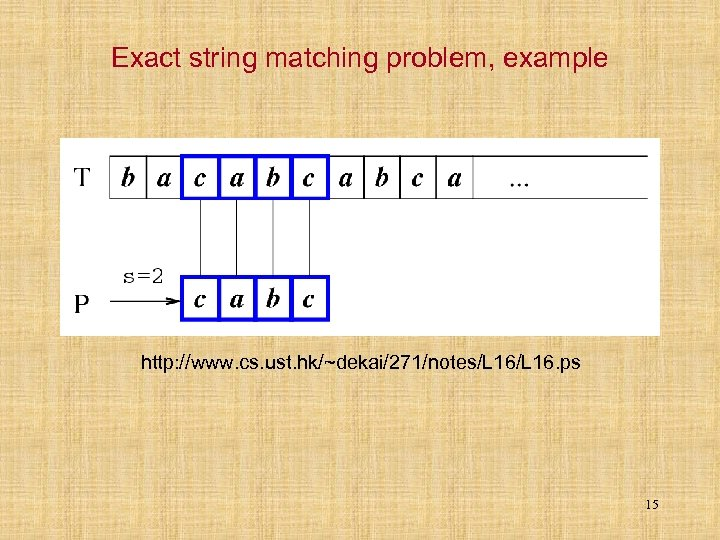Exact string matching problem, example http: //www. cs. ust. hk/~dekai/271/notes/L 16. ps 15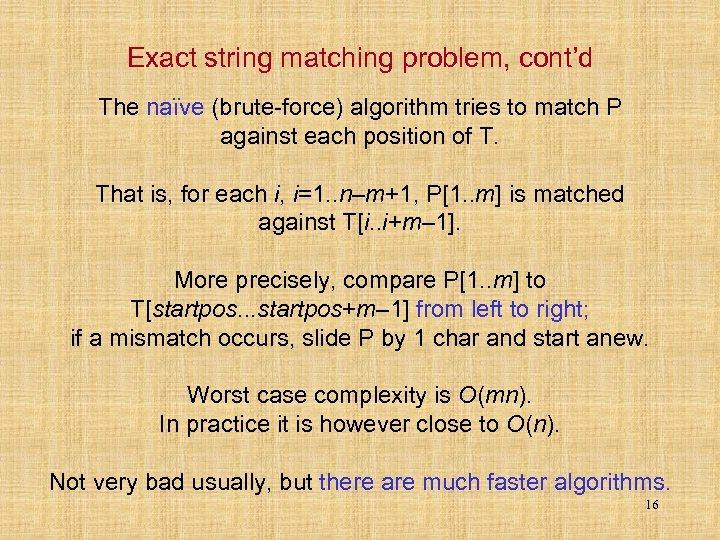Exact string matching problem, cont’d The naïve (brute-force) algorithm tries to match P against each position of T. That is, for each i, i=1. . n–m+1, P[1. . m] is matched against T[i. . i+m– 1]. More precisely, compare P[1. . m] to T[startpos. . . startpos+m– 1] from left to right; if a mismatch occurs, slide P by 1 char and start anew. Worst case complexity is O(mn). In practice it is however close to O(n). Not very bad usually, but there are much faster algorithms. 16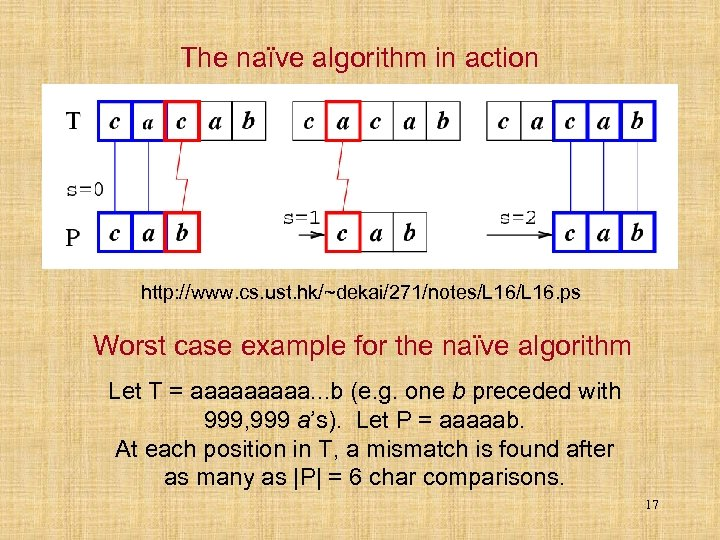The naïve algorithm in action http: //www. cs. ust. hk/~dekai/271/notes/L 16. ps Worst case example for the naïve algorithm Let T = aaaaa. . . b (e. g. one b preceded with 999, 999 a’s). Let P = aaaaab. At each position in T, a mismatch is found after as many as |P| = 6 char comparisons. 17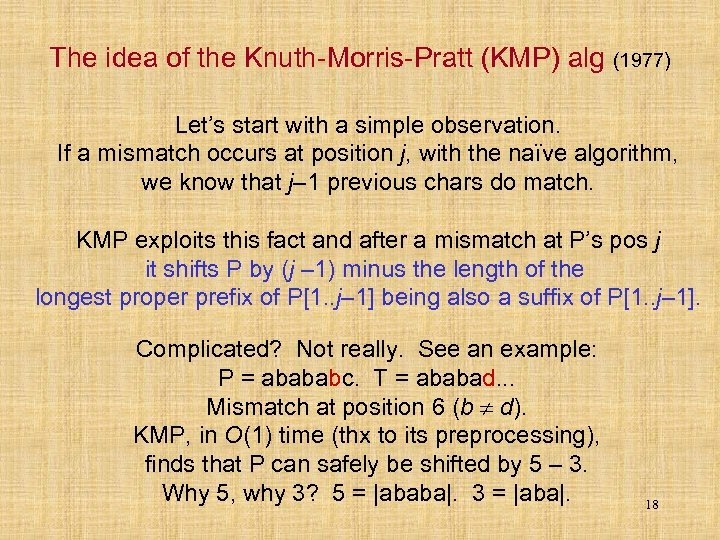The idea of the Knuth-Morris-Pratt (KMP) alg (1977) Let’s start with a simple observation. If a mismatch occurs at position j, with the naïve algorithm, we know that j– 1 previous chars do match. KMP exploits this fact and after a mismatch at P’s pos j it shifts P by (j – 1) minus the length of the longest proper prefix of P[1. . j– 1] being also a suffix of P[1. . j– 1]. Complicated? Not really. See an example: P = abababc. T = ababad. . . Mismatch at position 6 (b d). KMP, in O(1) time (thx to its preprocessing), finds that P can safely be shifted by 5 – 3. Why 5, why 3? 5 = |ababa|. 3 = |aba|. 18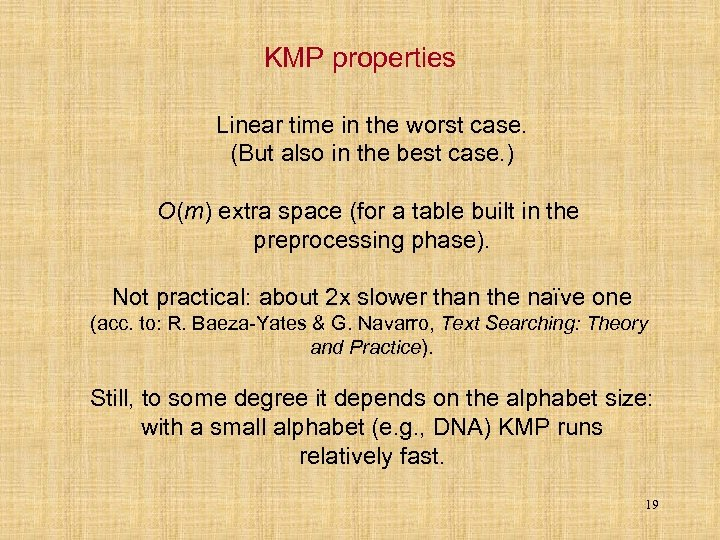KMP properties Linear time in the worst case. (But also in the best case. ) O(m) extra space (for a table built in the preprocessing phase). Not practical: about 2 x slower than the naïve one (acc. to: R. Baeza-Yates & G. Navarro, Text Searching: Theory and Practice). Still, to some degree it depends on the alphabet size: with a small alphabet (e. g. , DNA) KMP runs relatively fast. 19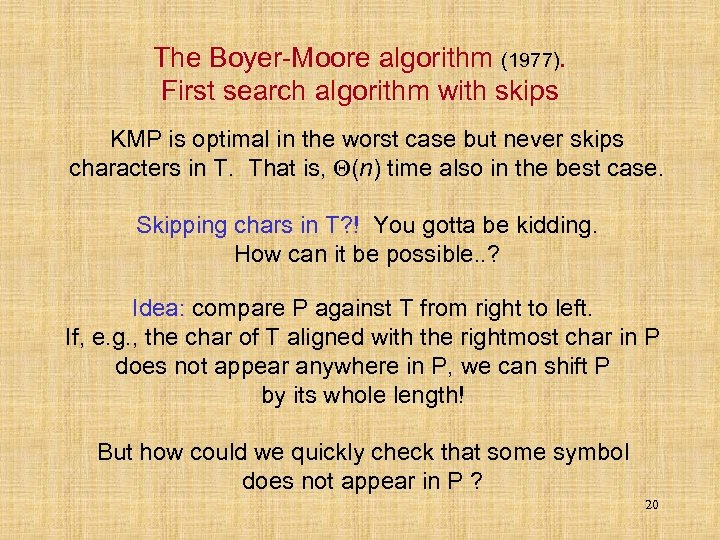The Boyer-Moore algorithm (1977). First search algorithm with skips KMP is optimal in the worst case but never skips characters in T. That is, (n) time also in the best case. Skipping chars in T? ! You gotta be kidding. How can it be possible. . ? Idea: compare P against T from right to left. If, e. g. , the char of T aligned with the rightmost char in P does not appear anywhere in P, we can shift P by its whole length! But how could we quickly check that some symbol does not appear in P ? 20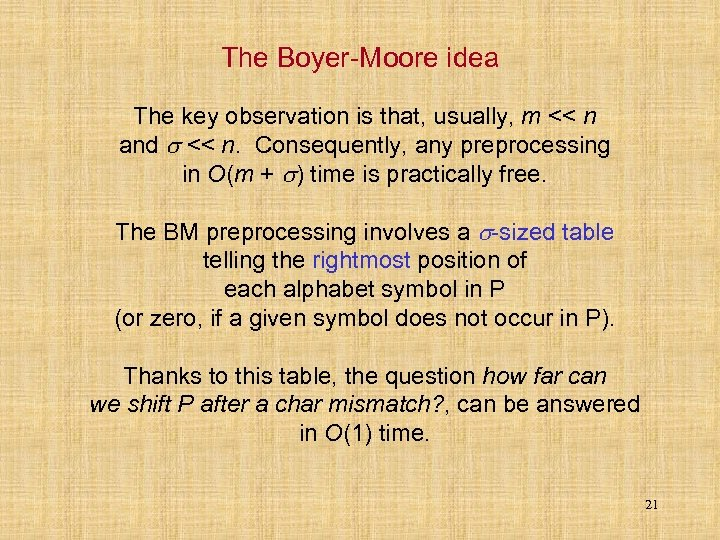The Boyer-Moore idea The key observation is that, usually, m << n and << n. Consequently, any preprocessing in O(m + ) time is practically free. The BM preprocessing involves a -sized table telling the rightmost position of each alphabet symbol in P (or zero, if a given symbol does not occur in P). Thanks to this table, the question how far can we shift P after a char mismatch? , can be answered in O(1) time. 21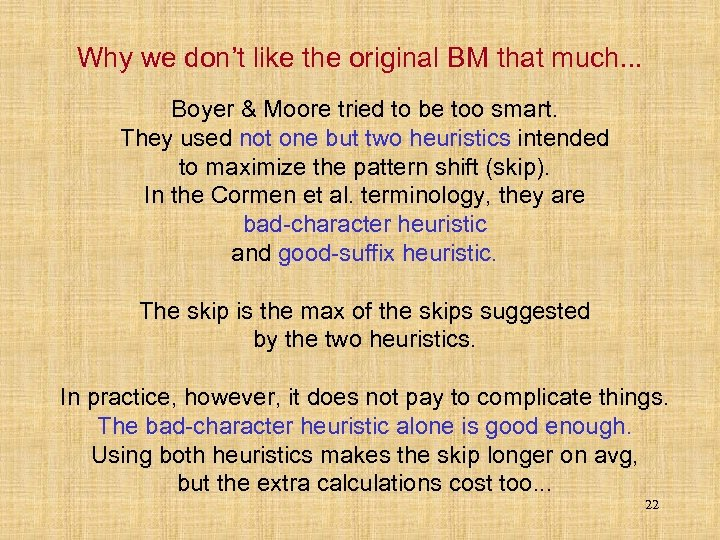Why we don’t like the original BM that much. . . Boyer & Moore tried to be too smart. They used not one but two heuristics intended to maximize the pattern shift (skip). In the Cormen et al. terminology, they are bad-character heuristic and good-suffix heuristic. The skip is the max of the skips suggested by the two heuristics. In practice, however, it does not pay to complicate things. The bad-character heuristic alone is good enough. Using both heuristics makes the skip longer on avg, but the extra calculations cost too. . . 22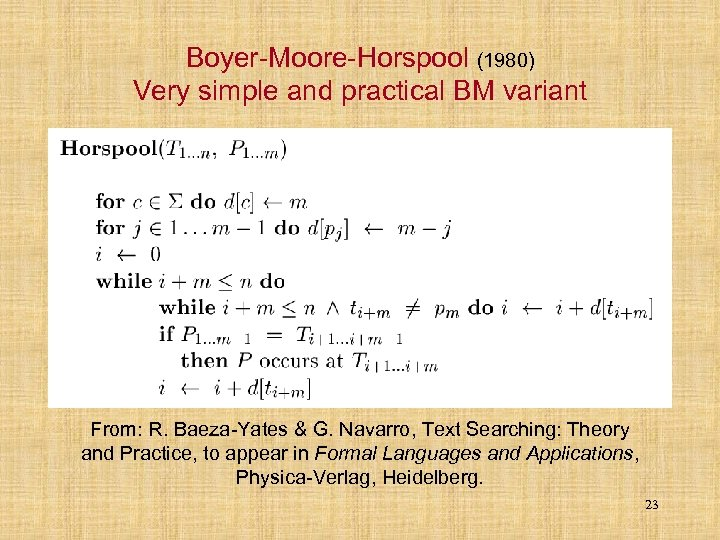Boyer-Moore-Horspool (1980) Very simple and practical BM variant From: R. Baeza-Yates & G. Navarro, Text Searching: Theory and Practice, to appear in Formal Languages and Applications, Physica-Verlag, Heidelberg. 23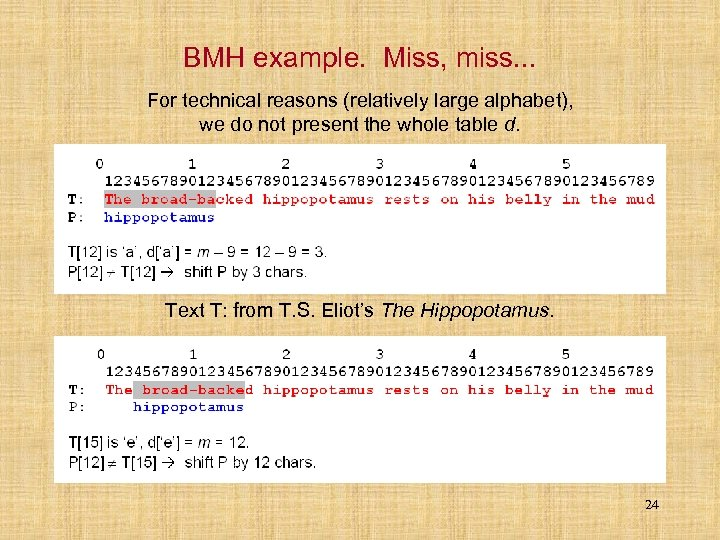BMH example. Miss, miss. . . For technical reasons (relatively large alphabet), we do not present the whole table d. Text T: from T. S. Eliot’s The Hippopotamus. 24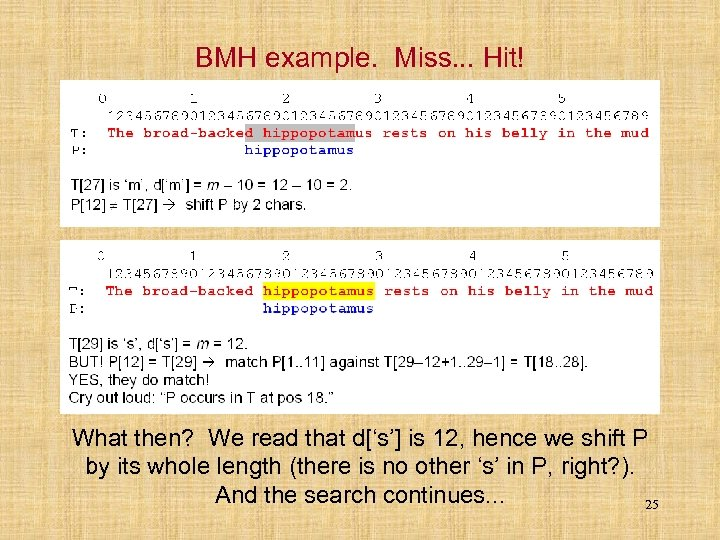BMH example. Miss. . . Hit! What then? We read that d[‘s’] is 12, hence we shift P by its whole length (there is no other ‘s’ in P, right? ). And the search continues. . . 25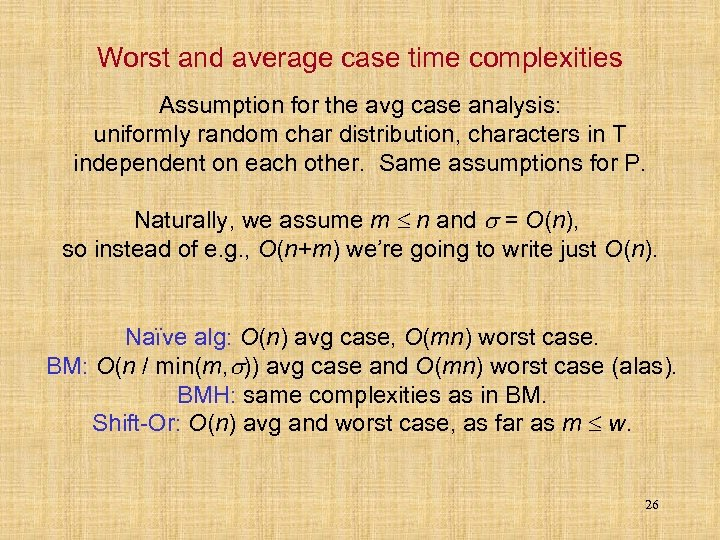Worst and average case time complexities Assumption for the avg case analysis: uniformly random char distribution, characters in T independent on each other. Same assumptions for P. Naturally, we assume m n and = O(n), so instead of e. g. , O(n+m) we’re going to write just O(n). Naïve alg: O(n) avg case, O(mn) worst case. BM: O(n / min(m, )) avg case and O(mn) worst case (alas). BMH: same complexities as in BM. Shift-Or: O(n) avg and worst case, as far as m w. 26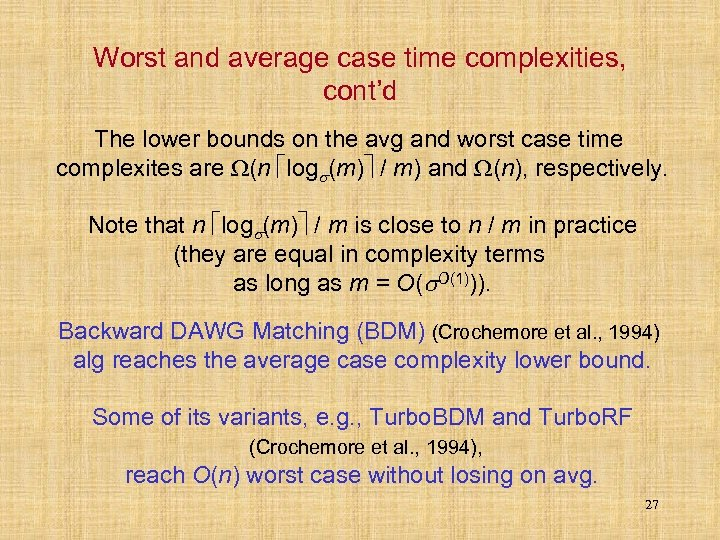Worst and average case time complexities, cont’d The lower bounds on the avg and worst case time complexites are (n log (m) / m) and (n), respectively. Note that n log (m) / m is close to n / m in practice (they are equal in complexity terms as long as m = O( O(1))). Backward DAWG Matching (BDM) (Crochemore et al. , 1994) alg reaches the average case complexity lower bound. Some of its variants, e. g. , Turbo. BDM and Turbo. RF (Crochemore et al. , 1994), reach O(n) worst case without losing on avg. 27